﻿ All the formula related to Alternating Current

# List of formulas related to alternating current

i: instantaneous current (A),

Io: maximum instantaneous current (A),

T: period of the wave,

f: frequency,

λ: wavelength,

c: speed of light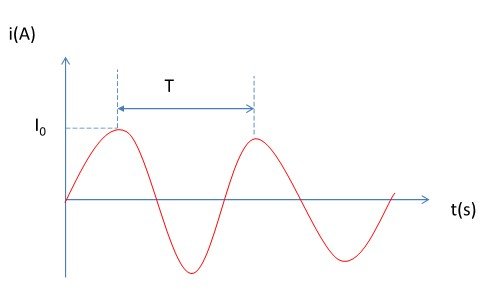$$i = i_o cos\omega t$$ $$f = \frac{1}{T}$$ $$\omega = 2\pi f$$ $$\lambda = \frac{c}{f} = cT$$ $$c \thickapprox 3 \text{ x } 10^8 m/s$$

$$U = \frac{U_o}{\sqrt{2}}$$ $$I = \frac{I_o}{\sqrt{2}}$$

$$U_R = IR$$ $$u_R = I_o R cos\omega t$$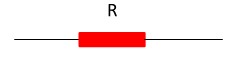ZL: inductive reactance

$$u_L = I_o Z_L cos(\omega t + \frac{\pi}{2})$$ $$Z_L = L_\omega$$ $$U_L = IZ_L$$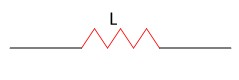ZC: capacitive reactance

$$u_c = I_o Z_c cos(\omega t - \frac{\pi}{2})$$ $$Z_c = \frac{1}{\omega C }$$ $$U_c = IZ_c$$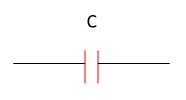Z: impedance

$$u = I_oZcos(\omega t + \phi)$$ $$Z = \sqrt{R^2 + (Z_L - Z_C)^2}$$ $$U = \sqrt{{U_{R}} ^2 + (U_L - U_C)^2}$$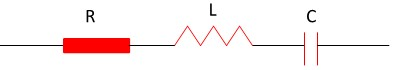$$tan\phi = \frac{U_L - U_C}{U_R}$$ $$= \frac{Z_L - Z_C}{Z}$$

$$cos\phi = \frac{U_R}{U} = \frac{R}{Z}$$

$$P = UIcos\phi = RI^2$$

$$\phi = 0$$ $$Z_{min} = R$$ $$I_{max} = \frac{U}{R}$$ $$Z_L = Z_C$$ $$\omega = \frac{1}{\sqrt{LC}}$$ $$cos\phi _{max} = 1$$ $$U_L = U_C$$ $$U = U_R$$

N1, N2: number of turns in the primary coil and secondary coil; U1, U2: primary and secondary voltage; I1, I2: primary and secondary current

$$\frac{U_1}{U_2} = \frac{N_1}{N_2} = \frac{I_1}{I_2}$$